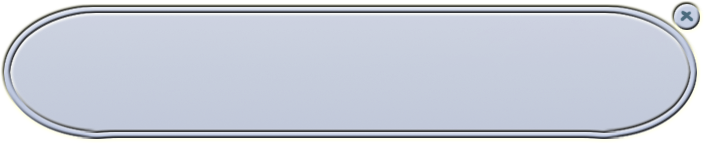krkr基础教程3

## 显示文字

[deffont face="黑体" size=20 color=0xffffff]

[defstyle linespacing=10 pitch=0 linesize=4]

[delay speed=10]

[font face="黑体" size=18 color=0xffffff edge=true edgecolor=0x787878]

[style linespacing=15 pitch=7 linesize=20]

1.第一次使用一个message的时候，一定要先用position设定这个图层的属性，而且要同时考虑到它的fore和back的情况
2.要写字或者要放按钮前，一定要先将焦点current到要放字或者按钮的图层

position是message设定的指令，用它可以设定message层的大小，位置，文字出现的位置，背景色或者背景图片等等，一个图层在使用前无论如何也要先用position设定为自己想要的样式才行，因为除了message0外，其他图层在在没设定的时候，长的都是一个纯黑色的15*15的小方框（layer也是），所以如果你在画面左上角看到一个这样的方块，说明你把什么图层没设定就visible=true了。
current是确定焦点的指令，它对焦上图层之后，后边的文字和按钮就都是放在那个图层上了，如果游戏一开始不写current就写文字或者按钮，他们会被放在message0上，如果在游戏中间，天知道你都做了什么操作之后，不写current就直接写文字或按钮，那么就只有神才知道那些东西被放在哪一层了……所以，在写文字和按钮的前边写current是非常必要的，注意trans之后也要重新current，不然也容易出错。[position layer=message0 left=50 top=430 width=704 height=140 marginl=71 margint=17 marginr=73 marginb=17 opacity=0 visible=true frame="frame" page=fore]
[current layer=message0 pgae=fore]
[deffont face="黑体" size=20 color=0xffffff]
[defstyle linespacing=10 pitch=0 linesize=4]
[er]

[font face="黑体" size=18 color=0x000000 edge=true edgecolor=0xffffff][style linespacing=15 pitch=7 linesize=20 align="center"]

［ｅｒ］之后［ｆｏｎｔ］和［ｓｔｙｌｅ］的设定就不管用了。

[position layer=message0 left=50 top=430 width=704 height=140 marginl=71 margint=17 marginr=73 marginb=17 opacity=0 visible=true frame="frame" page=fore vertical=true]
[current layer=message0 pgae=fore]
[deffont face="黑体" size=20 color=0xffffff]
[defstyle linespacing=10 pitch=0 linesize=4]
[er]

1.?[l]等待点击
2.?[r]位置换行，就是说后边的文字或者按钮会从当前行的下一行开始，行间距是开始的defstyle设定的那些数值
3.?[p]翻页，值得注意的是，KAGeXpress的[p]还承担着即时存档的功能，也就是存档的话，会存在[p]的位置，这是因为[p]在Conductor里被跳到了autolabel.ks的*autoLabelLabel标签，又跳了回来，于是[p]其实可以看做是一个翻了页然后标签了一下的东西，因为kr的存档只能呢个存在有标签的地方，于是在对话里需要靠[p]达成即时存档的效果
4.?[er]清空当前层上的内容，不改变用position设定的信息
5.?[cm]清空所有层，包括对所有层的position设定信息
6.?[ct]清空所有层，但是不改变已用position设定的信息
7.?[nowait]取消文字一个字一个字往外蹦的效果，一下子显示，相当于把文字速度设定为0，在遇到[endnowait]之前文字都会立刻显示
8.?[endnowait]结束[nowait]效果的指令

[position layer=message0 left=50 top=430 width=704 height=140 marginl=71 margint=17 marginr=73 marginb=17 opacity=0 visible=true frame="frame" page=fore]
[current layer=message0 pgae=fore]
[deffont face="黑体" size=20 color=0xffffff]
[defstyle linespacing=10 pitch=0 linesize=4]
[er]
[emb exp="‘今天天气真好'"]

[position layer=message0 left=50 top=430 width=704 height=140 marginl=71 margint=17 marginr=73 marginb=17 opacity=0 visible=true frame="frame" page=fore]
[current layer=message0 pgae=fore]
[deffont face="黑体" size=20 color=0xffffff]
[defstyle linespacing=10 pitch=0 linesize=4]
[er]

[eval exp="f.主角姓名='水螅'"]

[position layer=message0 left=50 top=430 width=704 height=140 marginl=71 margint=17 marginr=73 marginb=17 opacity=0 visible=true frame="frame" page=fore]
[current layer=message0 pgae=fore]
[deffont face="黑体" size=20 color=0xffffff]
[defstyle linespacing=10 pitch=0 linesize=4]
[er]

[emb exp="'哦呵呵呵呵'"][ch text="哦呵呵呵呵"]的区别在于ch不会自动换行，emb能够自动换行

[position layer=message0 left=50 top=430 width=704 height=140 marginl=71 margint=17 marginr=73 marginb=17 opacity=0 visible=true frame="frame" page=fore vertical=true]
[current layer=message0 pgae=fore]
[deffont face="黑体" size=20 color=0xffffff]
[defstyle linespacing=10 pitch=0 linesize=4]
[er]

deffont参数详解
size

face

color

rubysize

rubyoffset

edge

edgecolor

bold

defstyle参数详解
linespacing

pitch

linesize

delay参数详解
speed

font参数详解
size

face

color

rubysize

rubyoffset

edge

edgecolor

bold

italic

style参数详解
linespacing

pitch

linesize

align

autoreturn
true的话就是自动换行，默认true

position参数详解
layer

page

left

top

width

height

frame

framekey
0xRRGGBB格式，指定背景图片的透明色，对于直接用有通道的png图片的我来说……这个参数我从来没用过……
color

opacity

marginl

margint

marginr

marginb

vertical

visible

current参数详解
layer

page

withback

hydrozoa

2015-03-06 05:43:26

2015-02-15 20:46:26

hydrozoa

2014-11-16 07:33:25

2014-10-28 12:49:31

[position layer=message0 left=50 top=430 width=704 height=140 marginl=71 margint=17 marginr=73 marginb=17 opacity=0 visible=true frame="frame" page=fore vertical=true]
[current layer=message0 pgae=fore]
[deffont face="黑体" size=20 color=0xffffff]
[defstyle linespacing=10 pitch=0 linesize=4]
[er]

aa